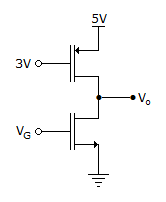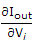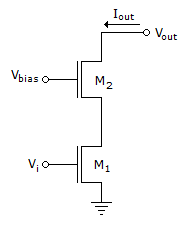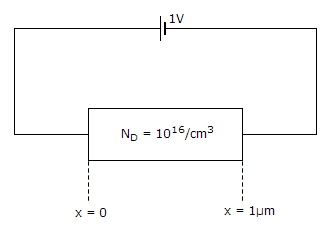# Electronics and Communication Engineering - Exam Questions Papers

36.

Consider the CMOS circuit shown, where the gate voltage VG of the n-MOSEFT is increased from zero, while the gate voltage of the p-MOSFET is kept constant at 3 V. Assume that, for both transistor, the magnitude of the threshold voltage is 1 V and the product of the transconductor parameter and the (W/L) ratio, i.e. the quality μC0x(W/L), is 1mA. V-2.For small increase in VG beyond IV, which of the following gives the correct description of the region of operation of each MOSFET?

 A. Both the MOSFET are in saturation region B. Both the MOSFET are in triode region C. n-MOSFET is in triode and p-MOSFET is in saturation region D. n-MOSFET is in saturation and p-MOSFET is in triode region

Answer: Option A

Explanation:

No answer description available for this question. Let us discuss.

37.

Two identical NMOS transistors M1 and M2 are connected as shown below. Vbias is chosen so that both transistors are in saturation. The equivalent gm of the pair is defined to beat constant Vout
The equivalent gm of the pair isA. the sum of individual gm's of the transistors B. the product of individual gm's of the transistors C. nearly equal to the gm of M1 D. nearly equal to gm / g0 of M2

Answer: Option C

Explanation:

No answer description available for this question. Let us discuss.

38.

In a digital voltmeter the oscillator frequency is 400 kHz and the ramp voltage falls from 8 V to 0 V in 20 ms. What is the number of pulses counted by the counter?

 A. 800 B. 2000 C. 4000 D. 8000

Answer: Option D

Explanation:

No answer description available for this question. Let us discuss.

39.

The silicon sample with unit cross-sectional area shown below is in thermal equilibrium. The following information is given: T = 300 K, electronic charge = 1.6 x 10-19C, thermal voltage = 26mV and electron mobility = 1350 cm2/V-sThe magnitude of the electron drift current density at x = 0.5 μm is

 A. 2.16 x 104 A/cm2 B. 1.08 x 104 A/cm2 C. 4.32 x 103 A/cm2 D. 6.48 x 102 A/cm2

Answer: Option A

Explanation:

No answer description available for this question. Let us discuss.

40.

Which of the following antennas uses a number of varying length parallel elements?

 A. Helical antenna B. Pyramidal Horn C. Comer reflection antenna D. Yagi-Uda antenna

Answer: Option D

Explanation:

No answer description available for this question. Let us discuss.

#### Current Affairs 2021

Interview Questions and Answers